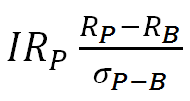# What is Information Ratio?

The information ratio is a commonly used risk-adjusted performance measure along with the Sharpe ratio. Even though both Information ratio and Sharpe ratio measure performance as a ratio of returns to the standard deviation in some form, there are some differences.

Sharpe ratio is the ratio of excess returns over risk-free returns to the total risk of the portfolio. Information ratio is the ratio of only the alpha component of the returns to the standard deviation of these excess alpha returns. It is calculated as the portfolio’s mean excess returns over a benchmark divided by the standard deviation of the excess returns.Where. RP represents the returns from the portfolio RB represents the returns from the benchmark σP-B represents the standard deviation of excess returns

The alpha component or the excess returns are the returns that can be attributed to the manager’s skill. The ratio only computes the returns per unit of risk attributable to the alpha component. For this reason, the ratio is widely used for measuring and comparing the performance of the portfolio managers. It can be used to tell if the manager outperformed the benchmark after adjusting for risk. However, it cannot really tell how exactly the portfolio outperformed the benchmark.

Note that high returns don’t necessarily mean high information ratio (IR). One can achieve higher IR by having a combination of high portfolio returns, low benchmark returns, and low tracking error (standard deviation of excess returns).

Let’s say that we have two portfolio managers John and Rob. John’s portfolio earns 15% returns while Rob’s portfolio earns 11%. However, the tracking error for John’s portfolio is 10% while for Rob’s portfolio it is only 5%. Both have the same index as the benchmark which has provided returns of 6%. The information ratio of both managers is calculated below:

IR of John = (15-6)/10 = 0.9 IR of Rob = (11 - 6)/5 = 1

Even though Rob’s portfolio has lower returns, it has higher Information ratio. A higher ratio indicates that on a risk-adjusted basis Rob has produced better returns.